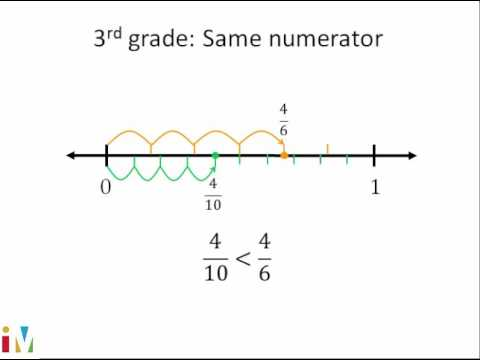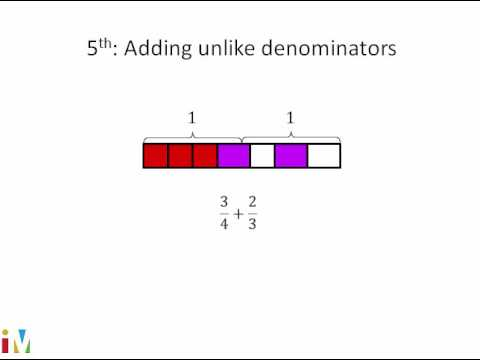Video

# Multiplying fractions and whole numbers visually (Full video)

Khan Academy

## Supporting grades: 4

Description: Sal gives a visual explanation of multiplying a fraction and a whole number. We've already seen that the fraction 2/5, or fractions like the fraction 2/5, can be literally represented as 2 times 1/5, which is the same thing, which is equal to literally having two 1/5s. And if we wanted to visualize it, let me make a hole here and divide it into five equal sections. So you could view this literally as being equal to 3 times 2 is, of course, 6, so this is the same thing as 6 times 1/5.

You must log inorsign upif you want to:*

*Teacher Advisor is 100% free.

### Other videos you might be interested in### Comparing Fractions

#### Illustrative Mathematics